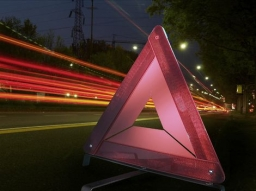# Circumference 43531

A triangle with a circumference of 69 cm has one side three times shorter than the longest of them and the other 3 cm shorter than the longest of them. Find the area of the triangle.

S =  142.1818 cm2

### Step-by-step explanation:

Try calculation via our triangle calculator.Did you find an error or inaccuracy? Feel free to write us. Thank you!

Tips for related online calculators
Do you have a linear equation or system of equations and looking for its solution? Or do you have a quadratic equation?